Statistical and Mathematical Concepts and Principles Applicable to Uncertainty Calculations for the Measurement of In Vivo Bone Lead by 109Cd K X Ray Fluorescence
Journal of Biometrics & Biostatistics

Like us on:

# Statistical and Mathematical Concepts and Principles Applicable to Uncertainty Calculations for the Measurement of In Vivo Bone Lead by 109Cd K X Ray Fluorescence

José A. A. de Brito*

Instituto Superior de Ciências da Saúde Egas Moniz, Campus da Granja, Monte de Caparica, 2829-511 Caparica, Portugal

*Corresponding Author:
José A. A. de Brito
Instituto Superior de Ciências da Saúde Egas Moniz
Campus da Granja, Monte de Caparica
2829-511 Caparica, Portugal
E-mail: [email protected]

Received Date: November 25, 2011; Accepted Date: January 12, 2012; Published Date: January 16, 2012

Citation: de Brito JAA (2012) Statistical and Mathematical Concepts and Principles Applicable to Uncertainty Calculations for the Measurement of in vivo Bone Lead by 109cd K X Ray Fluorescence. J Biom Biostat 3:133. doi:10.4172/2155-6180.1000133

Copyright: © 2012 de Brito JAA. This is an open-access article distributed under the terms of the Creative Commons Attribution License, which permits unrestricted use, distribution, and reproduction in any medium, provided the original author and source are credited.

Visit for more related articles at Journal of Biometrics & Biostatistics

#### Abstract

This work discusses the statistical and mathematical concepts and principles applicable to in vivo 109Cd K X Ray Fluorescence measurements (109Cd KXRF) of lead in human bone. The primary aim of this paper is to examine divergent views about the quantitative methods applied to estimate in vivo bone lead concentrations and their uncertainty when using the 109Cd KXRF technique. The emphasis is on the potential effect of covariance between quantities of interest on the result of an in vivo measurement of bone lead by 109Cd KXRF, a question which has received little attention in view of the long standing problem of why the average measurement uncertainty is less than the standard deviation of repeated measurements.

#### Keywords

Bone lead; in vivo 109Cd KXRF; Measurement uncertainty

#### Introduction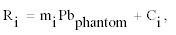(1.1)

where i = a, b; Ri = xi/coh; and mi and Ci are, respectively, the slope and the intercept of the calibration line derived by least squares. One should note that the peak amplitudes estimated by the Marquardt method are estimates of the mean peak amplitude, that is, the most probable value under the assumption of normality, if the measurements were repeated many times . Following the general theory of calibration, each line is corrected for prediction of the in vivo concentrations, which is calculated as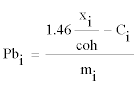(1.2)

where constant 1.46 is the ratio of coherent scattering cross section of plaster of Paris to bone mineral at 88 keV and 153°. The current method reports the result of a 109Cd KXRF bone lead measurement as the inverse variance weighted mean (Pbm) of the peak concentrations Pbα and Pbβ, that is,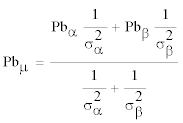(1.3)

where quantities σα and σβ are the uncertainties in the bone lead peak concentrations calculated from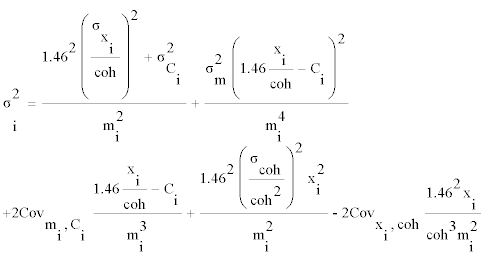(1.4)

where covxi,coh is forced to zero [3,5,6]. In the current method, this covariance is forced to zero because it is considered that the two peak amplitudes are independent, as they originate from two distinct physical processes, despite the claim that there are situations in which a correlation exists between them [7,8]. The robustness of this assumption, however, is contradicted by data showing the existence of significant correlations between the lead and coherent peak amplitudes, as well as by the nature of the mechanisms of interaction between gamma rays and matter, which shall be addressed later.

Following (1.3), the current method estimates the uncertainty of the inverse variance weighted mean bone lead concentration as the square root of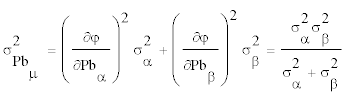(1.5)

which relies on the assumption that the fluctuations in the measurement of the two peak concentrations are uncorrelated. Alternatively, Brito  has proposed that the measurement uncertainty be estimated using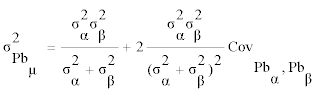(1.6)

where the covariance term is estimated from the correlation coefficient between the two peak concentrations, following the known relationship between covariance and correlation: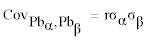(1.7)

It is commonly believed that the uncertainty in a bone lead concentration assessed by 109Cd KXRF is an estimate of the standard deviation of replicate measurements , but it has been noted that the measurement uncertainty underestimates the standard deviation of repeated measurements by 20 to 50% [3,10]. In this context, the revision proposed by Brito  for the calculation of overall measurement uncertainty has shown that the uncertainties in bone lead concentrations may have been underestimated by the current method by as much as 38 – 40%. That revision seems to help reduce the abovementioned discrepancies between bone lead concentration uncertainty and standard deviation of replicate bone lead measurements, but the approach proposed by Brito  has been challenged by O’Meara and Fleming , on the grounds that it relies on an ill-defined and unexpectedly large correlation coefficient.

#### Covariance between the Lead and Coherent Peak Amplitudes

Statistical concepts and principles

Before addressing the opposite views of the problem, and in order to remove any obscurity arising from terminology, in the remaining part of this paper peak amplitudes and their ratio are treated as random variables and not as mathematical variables in the ordinary sense. Otherwise, it would be statistical nonsense to speak of their distribution and even more of their variance, covariance or correlation. Therefore, as random variables, nothing precludes the assessment of the correlation or covariance between the lead and coherent peak amplitudes .

Although the predominant view in the field of in vivo 109Cd XRF of lead in bone is that such covariance does not exist, only O’Meara and Fleming  provide arguments in support of such view. According to those authors, the covariance between peak amplitudes is zero because the peak amplitudes originate from distinct physical processes. This requires clarification, because the fact that those processes are distinct is not a sufficient condition for uncorrelation or zero covariance between the random variables originated by them, as shown by many examples in the literature pertinent to in vivo 109Cd KXRF of lead in bone. For instance, the physical processes that govern the build up of lead in bone, originating the bone lead concentration, are distinct from those governing the binding of lead to the red blood cells, which are at the origin of the cumulative blood lead concentration. Nevertheless, a non- zero covariance exists between those two concentrations, as expressed by the significantly large correlations reported in the literature by Roels et al.  and Fleming et al. . In addition, to explain why there is no covariance between the peak amplitudes, O’Meara and Fleming  also state that those variables are independent, although they do not explain whether it refers to the absence of a causal or functional relationship between the variables or to statistical independence between them. In any case, this warrants further explanation.

In fact, the absence of causal or functional relationships between two variables does not preclude the assessment of the magnitude and significance of the correlation between them, provided that one does not incur in the logical fallacy that correlation implies causation or uses the regression line to express a functional or structural relationship between the variables [11,13]. Moreover, if the term independent is used in the statistical sense, then the argument is somewhat flawed with issues of consistency, in the light of a well known theorem: if two random variables X and Y are statistically independent, then their covariance is zero  which refutes the claim that, although the lead peak amplitude is independent of the coherent peak amplitude, there are situations in which a correlation exists between them.

A new perspective has been added to this discussion by O’Meara and Fleming , who claim that the correlation between the amplitudes of the two peaks should not be attributed to covariance between the terms because it merely indicates their mutual dependence on third variables. This warrants further explanation because there is a conceptual link between correlation and covariance, as explained in Statistics texts. For example, Mosteller and E.K.Rourke  simply define ρx,y, the Pearson correlation coefficient between X and Y, as ρX,Y = Cov(X´,Y´), where the second term is the covariance between X´ and Y´, the standardized random variables given by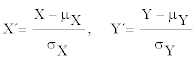(2.1)

Bevington and Robinson , instead, define ρX,Y and Cov(X,Y) from which one arrives at the following equation: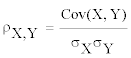(2.2)

To make this clearer, as measures of linearity between two variables, the two quantities are absolutely equivalent. In fact, the correlation coefficient is the covariance between standardized X and Y and it is used merely to overcome the dependence of covariance on the measurement units of X and Y and not to add any new dimension to the interpretation of correlation . Therefore, since no correlation can exist without a covariance between the variables involved, the suggestion by O’Meara and Fleming  that the equations used by Brito  imply an unexpected relationship between ρX,Y and Cov(X,Y) seems incomplete.

In any case, if by disentangling correlation from covariance O’Meara and Fleming  wish to remove any spuriousness in the relationship between peak amplitudes, due to mutual dependence on third causes, then their concern is unjustified. In fact, for the fathers of correlation and regression, such as Galton and Pearson cited by Aldrich : “the correlation between two variables measures the extent to which they are governed by common causes” (Galton); “the correlated observables are determined by a great variety of independent contributory causes” (Pearson). Therefore, there is nothing wrong in a correlation that emerges from mutual dependence on common causes, and there is no need to remove any spuriousness from the relationship between the two peak amplitudes. In fact, if there were such problem, then spurious correlations would also undermine a host of published work in the field of in vivo 109Cd KXRF. For example, correlation coefficients as high as 0.9 between bone lead concentration and cumulative blood lead concentration have been reported by Fleming et al.  which mainly reflect the mutual dependence of the two biological indexes on exposure time more than on anything else.

The photon counting process in 109Cd KXRF

In order to overcome the insufficiency of current explanations for the covariance structure of the photon counting process in the 109Cd KXRF technique, and to demonstrate that the peak amplitudes are not statistically independent, a brief discussion of the interaction mechanisms for gamma rays in matter is provided now.

Although there are many possible interaction mechanisms for gamma rays in matter, only three major types are important for the low gamma ray energy used in the 109Cd KXRF technique: photoelectric absorption, Compton scattering and coherent scattering . The cross section for each of these processes depends on the atomic number of the absorber and incident energy; for Compton and coherent interactions, it also depends on the angle of scatter [1,4]. Therefore, for the adopted measurement geometry (180°) and excitation energy (88.035 keV) used in the 109Cd KXRF technique, it is possible to assign a probability value to each of the possible outcomes of the interaction between the incident gamma rays and the sample material. Of course, there is also a non-zero probability that the incoming photons do not interact at all with the sample material.

In these conditions, let N be the total number of 88.035 keV photons emitted by the 109Cd source, which enter the sample volume viewed by the detector during a 109Cd KXRF measurement in a pre-defined acquisition time. For each of these photons, there are four possible, mutually exclusive, outcomes in the sample material: photoelectric absorption, Compton scattering, coherent scattering or no interaction at all, which occur with probabilities pX, pC, pcoh and p∅, respectively. If X, C, coh and ∅ denote the number of times those outcomes, respectively, occur in the sample during the measurement, then the random vector (X, C, coh, ∅) has a multinomial distribution.

Any considerations on the mean, variance and covariance for each pair of X, C, coh and ∅ rely on the assumption that the values of those variables change from one sample of exactly N incoming photons to another. According to Mosteller and Rourke , the expected value (E) and variance (Var) of X, C, coh and ∅ are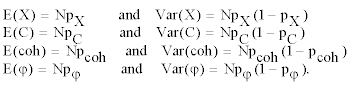(2.3)

To study the degree to which any two of the X, C, coh and ∅ variables vary together from a sample of exactly N incoming photons to another, one needs to know the covariance between those counting variables. For the particular case of photoelectric absorption and coherent scattering, the covariance and correlation are given by Cov(X,coh) = - NpX pcoh and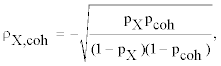(2.4)

respectively, and similarly for any pair of outcomes.

Therefore, if it were possible to repeat the measurements with all conditions held constant, including the total number of incoming photons, then one would expect an inverse relationship between the number of photoelectric absorption and coherent scattering events occurred during the measurement. This is a clear indication of statistical dependence between these two variables, even in the case where all the things which they depend on, such as acquisition time, source-sample distance, and source activity are held constant. Clearly, this refutes the dominant view in the field of in vivo 109Cd KXRF of lead in bone, that there is no covariance between the amplitudes of the corresponding peaks.

Furthermore, as expected from the discussion of the multinomial distribution, any experimental factor that makes the number of incoming photons (N) to vary between measurements will introduce a mutual dependence between the expected values of X and coh in each measurement. Examples of such factors are the source activity and, mainly, the source-sample distance, as clearly demonstrated by Somervaille et al. . Therefore, since E(X) and E(coh) have a mutual dependence on the number of incoming photons, there is a functional relationship between those quantities, given by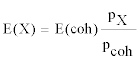(2.5)

In view of this functional relationship, a strong and positive correlation is expected between experimental data on the two quantities, E(X) and E(coh), which has been observed in independent work by Brito  and Rebôcho et al. . Thus, far from being a singularity, as suggested by O’Meara and Fleming , the high correlations between peak amplitudes observed by different authors in independent work are expected from theory.

Further investigations of covariance: experiments and data simulations

O’Meara and Fleming  also investigated the existence of a covariance between the lead and coherent peak amplitudes through experiments and simulation work described in detail in their paper. In short, the experiments consisted of a series of 20 repeated measurements performed with a 20 ppm plaster of Paris lead phantom, with all experimental variables held constant. The Monte Carlo work consisted of 25 simulations runs for a 20 ppm plaster of Paris phantom, also with all experimental variables held constant. From the description of the procedures provided by the authors, it is legitimate to admit that the measurements and the simulations did not account for phantom repositioning.

Their work is of limited external validity because it was performed under experimental conditions that are not those observed in field studies, such as the work of Brito , except for the acquisition time, which was constant in both cases. In effect, those authors performed:

- 20 repeat measurements with a 20 ppm plaster of Paris lead phantom, while Brito  performed 109 repeat measurements with a collection of plaster of Paris lead phantoms at 8 different concentrations (0 to 200 ppm);

- repeat measurements with the source-sample distance held constant, while in the study reported by Brito  the phantom measurements were interspersed with in situ and in vivo measurements, with sample repositioning at varying (not controlled) source-sample distance;

- repeat measurements over a short period (otherwise, the condition of a constant source activity does not hold), while the repeat measurements performed by Brito  took place over a period of two months, therefore the source activity was not constant.

Of course, in an in vivo bone lead survey it is not necessary to use exactly the same source-sample distance and source activity, as stated by O’Meara and Fleming . But that is a rhetorical question because, strictly speaking, it is impossible to comply with those requirements, particularly in the lead surveys conducted by Brito , in which 549 subjects had their bone lead concentrations measured on two bone sites during a 40-minute measurement over a period of two months, interspersed with more than a hundred measurements with standards. Although there was an effort to position the samples at 2 – 3 cm from the source, it is not realistic to expect that all samples were exactly at the same distance from the source during the measurements. Similarly, it is not realistic to assume that the 549 subjects were exactly at the same distance from the source and remained motionless during a 40-minute measurement. Therefore, a covariance between the measured lead and coherent peak amplitudes naturally arises in the measurement of both standards and subjects.

#### Alternative Approach to the Weighted Mean Lead Concentration

O’Meara and Fleming  recognize that the approaches to the error in the weighted mean lead concentration, performed independently by Brito  and Todd and Chettle  are mathematically correct, but they question the use of (1.7) above, on the grounds that it requires the use of an ill-defined parameter. Following the dominant view in the field, the authors base most of their alternative approach to the weighted mean lead concentration on the following three conditions: first, there are conditions that warrant the exclusion of the calibration line intercept; second, the Pearson correlation coefficient (r) between the lead peak concentrations is ill-defined and unexpectedly large; third, several random variables used in different equations in their paper have no covariance or are treated as constants. Unfortunately, these statements, if not actually errors or misconceptions, are open to debate.

Proposed treatment for calibration line intercepts

In their approach to the weighted mean lead concentration, O’Meara and Fleming  state that the proposed method will only apply in situations in which the intercept of the calibration line is not included in the calculation of the Pb concentration from the spectral data. According to the authors, this is warranted when the source of the intercept is well characterized and known to come from trace contamination by Pb in the plaster of Paris standards, as proposed by Todd . In that paper, it is explained the role played by the statistical significance of the calibration intercept in the proposed treatment to that parameter for the subtle intrinsic contamination scenario. In fact, the statistical significance of that parameter is used to define a gradient within the subtle contamination scenario. First, Todd  states: “Calibration line intercepts that do not differ significantly from zero would be obtained from plaster of Paris contaminated to an appropriately low (or zero) degree”. Then, the same author adds: “Positive calibration line intercepts that are significantly different from zero are the type observed most commonly. They suggest that the plaster of Paris is contaminated with trace levels of lead, as described above”.

That an appropriately low (or zero) degree of contamination is conceptually different from a trace level of contamination, seems clear. That this difference exists and has enough weight to determine different actions to treat the calibration intercepts, one for each degree of subtle contamination, as outlined by Todd  also seems clear. Consequently, issues of consistency may undermine the validity of the first of premises in the alternative approach proposed by O’Meara and Fleming .

In fact, in practical situations, there is a potential inconsistency resulting from the proposed treatment of calibration line intercepts outlined by Todd . For example, when the experimenter uses the same set of plaster of Paris standards to fit two different calibration lines (alpha and beta), it may happen that the intercepts indicate different degrees of contamination. Based on repeated measurements of the same set of standards, it is possible to derive calibration lines whose intercepts do not show the same level of significance: quite often, the alpha intercept is significantly positive, while the beta intercept is nonsignificant. According to Todd’s interpretation, this outcome would suggest that the standards used in that calibration were intercept) but also that the same standards were contaminated to an appropriately low (or zero) degree (if based on the significance of the beta intercept). This situation could result in an inconsistent treatment of the two intercepts.

A much more serious problem, though, is the mathematical treatment proposed by Todd  for the calibration line intercept, for the subtle (intrinsic) contamination scenario. The recommended action for the case of a positive significant intercept is addressed first, since it represents a considerable “bend” of classical regression. In fact, in the classical theory of calibration, Y (here, the ratio of lead to coherent amplitude) is regressed against X (here, the lead concentration in standards) and then X is solved for Y with the purpose of predicting X for a given value of Y . Alternatively, given that Y and X are highly correlated, inverse regression could be used to estimate X as a function of Y. In any case, though, the use of the intercept to correct the values of X in order to force the intercept to be zero within errors in a second iteration, which is the intermediate step to arrive at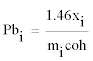(3.1)

in the paper by O’Meara and Fleming , is simply not an option available in this method. In the case of a non-significant intercept, the fitting of a calibration line passing through the origin is the treatment proposed by Todd . This, however, is in glaring contrast with the concerns expressed by many authors about the problems raised by the use of such model. Among them is the use of conventional statistics (for example, the R2 and model F), which are not comparable between the intercept and the no-intercept models, leading to erroneous conclusions [19,20]. Therefore, since one of the premises of the alternative approach proposed by O’Meara and Fleming  is clouded with issues of consistency and correctness, their results should be considered cautiously. Another reason for concern with the use of this alternative approach arises from considerations by the authors about the correlation coefficient between the lead peak concentrations, which are addressed in the next section.

Correlation coefficients between lead peak concentrations

Before presenting their alternative method, O’Meara and Fleming  question the legitimacy of calculating a weighted mean of correlated quantities and suggest that calculating the generalized weighted mean of the lead peak concentrations would yield a form of average that accounts for the covariance matrix of these concentrations. This statement is obscure and requires further considerations.

To begin, O’Meara and Fleming  vigorously question the use of (1.6) and (1.7) above, because this would require the Pearson correlation coefficient between lead peak concentrations and this coefficient, in their view, is ill-defined. However, the authors are not clear about which parameter, r or r2, they wish to prove is ill-defined, as can be concluded from the reading of their paper. Since what is at stake here is the concept of correlation (or covariance), as measured by the Pearson correlation coefficient r, and since there is no formal definition of r2 other than the obvious square of r, the following discussion assumes that the authors are focused on the ill-definition of r.

In their paper, the authors conclude that the Pearson correlation coefficient is ill-defined, based on arithmetical considerations on r2 values. These values are estimated from the correlation between lead peak concentrations, using their 20 experimental data points and two subsets of 15 of these points selected in a random fashion. The authors report r2values of 0.034 for the set of 20 points, 0.0028 for one subset of 15 points and 0.071 for the other subset. They claim that the Pearson correlation coefficient is ill-defined because these values differ by a factor of more than 25. However, nowhere in the paper do the authors provide any evidence that this difference reaches any statistical significance. Moreover, what the authors should have considered in their comparisons is the value of r, not r2, because their point is that the Pearson correlation coefficient r is ill-defined, not that r2 is illdefined. Had the authors done so, they would have concluded that the r values differ by a factor of 5, not 25. Second, the authors should have assessed the differences between the observed values of the correlation coefficients using a proper statistical test. Had they done so, they would have concluded that none of the r values calculated from their experiments, 0.1944, 0.0529 and 0.2665, can be distinguished from zero by a bilateral test of hypotheses at the 5% level of significance, as expressed by p values of 0.410, 0.8514 and 0.3370, respectively. Moreover, and as expected from these results, there are no significant differences between any two of the correlation coefficients observed by O’Meara and Fleming , as expressed by p values of 0.711, 0.832 and 0.588 estimated by a bilateral test of hypothesis applied at the 5% level of significance.

The authors’ attempt to force the existence of differences between correlation coefficients when there is none is particularly troubling. However, the most important flaw in the arguments presented by O’Meara and Fleming  against the use of the Pearson correlation coefficient proposed by Brito  resides in the fact that, according to the authors, it implies the use of excessively large values for r. In fact, close reading of published work would show that no less than a strong correlation should be expected between the two lead peak concentrations, as a result of the linear functional relationship that exists between the ratios Ri of lead (xi) to coherent (coh) peak amplitudes. This can be concluded from the following statement by Somervaille et al. : “Because the coherent scattering cross section is so flat in the backward direction, the ratio of (lead x-rays produced/γ rays coherently scattered towards the detector) is directly proportional to the ratio of (lead/bone mineral) at the measurement site, and is independent of position”.

In the statement above, no distinction is made regarding the ratios calculated for the Kα1 and the Kβ1 lead peaks, so it is legitimate to conclude that those ratios have a mutual linear functional dependence on the same quantity. Therefore, a significant correlation and, conceivably, large Pearson correlation coefficients between the lead peak concentrations are expected. This shows that the use of (1.6) and (1.7) presented in this paper, which were derived following a mathematically correct approach, as recognized by O’Meara and Fleming , is not hindered by any ill-condition affecting the Pearson correlation coefficient.

The weighted mean of correlated quantities

Before concluding, the formula used by O’Meara and Fleming  to estimate the covariance between lead peak concentrations is now addressed. In order to express such covariance between Pbα and Pbβ, those authors write the covariance as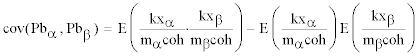(3.2)

where k = 1.46 and E is the mean of the quantity enclosed. Then the authors write the same covariance as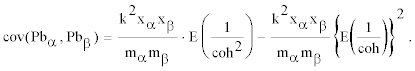(3.3)

The authors move from (3.2) to (3.3) considering k, xα, xβ, mα and mb to be constants. This is incorrect, because to do so would jeopardize the conceptual integrity of their paper. In effect, it is not immediately clear how peak amplitudes xα and xβ, which are random variables subject to variance, can also be treated as constants for the purpose of estimating the mean terms in (3.2) leading to (3.3). From this last equation, the authors arrive at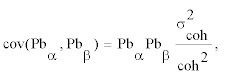(3.4)

an expression for covariance which is then incorporated in the following equation to give the uncertainty in the weighted mean lead concentration: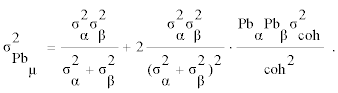(3.5)

This formula is obviously clouded with the issues of correctness mentioned above and, in contrast to what is argued by O’Meara and Fleming , it does not correctly describe the uncertainty in the weighted mean lead concentration. It is true that the authors propose an alternative approach to the weighted mean concentration, in order to avoid the use of an ill-defined correlation coefficient between the peak concentrations, but their approach is undermined by the use of a series of ill-conditioned quantities.

In fact, if xα, xβ, ma and mβ are constant, then their variances are zero. Consequently, the variances of Dα= xα/mα and Dβ = xβ/mβ are zero, and quantities Dμ and 2 σ2 , presented by O’Meara and Fleming  as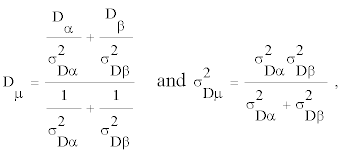(3.6)

are both undefined. As a result, the weighted mean lead concentration Pbμ and its variance σ2PBμ presented by the same authors as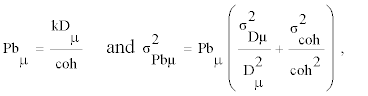(3.7)

are also undefined. In conclusion, if those four quantities are constant, then the alternative approach to the weighted mean of correlated quantities proposed by O’Meara and Fleming  is incorrect.

#### Discussion

Most of the criticism received by Brito  from O’Meara and Fleming  is at least open to debate, as the arguments presented by those authors appear to be clouded with issues of correctness in the use of basic statistical and mathematical concepts and principles. In addition, some of those arguments are contradicted by evidence provided by independent studies.

Their investigation of the existence of a covariance between the lead and coherent peak amplitudes through experiments and simulation work is of limited external validity, because it requires experimental conditions that are not those observed in field studies. In an in vivo bone lead survey it is impossible to comply with requirements such as a constant source-sample distance or constant source activity, and therefore, a covariance between the measured lead and coherent peak amplitudes naturally arises in the measurement of both standards and subjects. It should be noted that this covariance is genuine since it does not result from any mathematical procedure implemented by the researchers and which has been identified by many authors as sources of spurious correlations . Therefore, uncertainty calculations should not ignore this covariance, because it results from factors that also have an impact on the signal-to-background ratio and, therefore, on the measurement uncertainty. Furthermore, performing in vivo measurements with a system calibrated under conditions that are not those observed in situ and ignoring the observed covariance in phantom measurements, violates good laboratory practice and jeopardizes the internal validity of a measurement process.

This would be a minor problem if some of the arguments presented by O’Meara and Fleming . throughout their paper were not insufficient and incomplete quotes of published work. One example of this is the authors’ reference to the work of Somervaille et al.  in support of their claim that the normalization procedure used in the in vivo 109Cd KXRF method renders the measurement independent of variations in the source-sample distance, among other factors. Presented this way, the statement is both incomplete and misleading and should be clarified.

First, the result of a measurement is only an approximation or estimate of the value of the specific quantity subject to measurement, and thus the result is complete only when accompanied by a quantitative statement of its uncertainty . Second, what Somervaille et al.  have reported is that the ratio xi/coh between the lead and coherent peak amplitudes, and therefore the estimated bone lead concentration, is independent of all geometric effects including the source sampledistance, while the precision of the method, which is determined by the signal-to-background ratio, depends on the source-sample distance. To illustrate this, Somervaille et al.  even report an 18% decrease in the width of the Compton peak with an increase from 2 to 3 cm in the source-subject distance, regardless of any normalization. Furthermore, in direct contrast with suggestion by O’Meara and Fleming , it is not immediately clear how an arithmetical procedure performed a posteriori such as the normalization using coherently gamma rays would interfere with the physical processes that govern the signal-to-background ratio and, ultimately, the measurement uncertainty. Therefore, it is true that the ratio xi/coh provides a robust estimate of the lead concentration, but that is hardly the problem under discussion here. What is here at stake is measurement precision, largely dominated by quantities affected by the signal-to-background ratio and not by the ratio xi/coh, as suggested by O’Meara and Fleming .

Using the data provided by the same experiments and simulation work, O’Meara and Fleming  also question the use of (1.6) and (1.7) above, because this would require the use of an ill-defined and excessively large Pearson correlation coefficient between lead peak concentrations. First, the authors fail to provide any evidence that the numerical differences between the correlation coefficients estimated based on their experiments and simulations reach statistical significance. Therefore, if these data are all that is available for establishing that the Pearson correlation coefficient r is ill-defined, a major weakness arises in the arguments used by O’Meara and Fleming , because their data do not in the slightest indicate such illness. Actually, this particular interpretation of differences between correlation coefficients is also evident in the discussion section of their paper, when it is claimed that a correlation coefficient of 0.19, estimated in their experiments for the association between lead peak concentrations, is found to be well outside the range of 0.44 – 0.99 reported by Brito  for the same association. In the absence of any inferential test, these comparisons are totally useless.

Finally, the formula used by those authors to estimate the covariance between lead peak concentrations, and arrived at by the authors through derivations described in the Appendix of their paper, is clouded with issues of correctness which propagate into other equations and seriously jeopardize the alternative approach proposed by the authors to the weighted mean lead concentration.

As a suggestion, in future work these authors should explore the power of Monte Carlo simulations in a more realistic way, closer to the conditions encountered by the experimentalist, in situ and away from the ideal conditions of a lab. In so doing, and following an appropriate data analysis process, it is conceivable that most of the questions raised by Brito  will be properly addressed by those authors and will contribute to a better understanding of a long standing problem in the field of in vivo 109Cd KXRF of lead in bone.

#### References

Select your language of interest to view the total content in your interested language

### Article Usage

• Total views: 11945
• [From(publication date):
January-2012 - Dec 16, 2019]
• Breakdown by view type
• HTML page views : 8145Can't read the image? click here to refresh